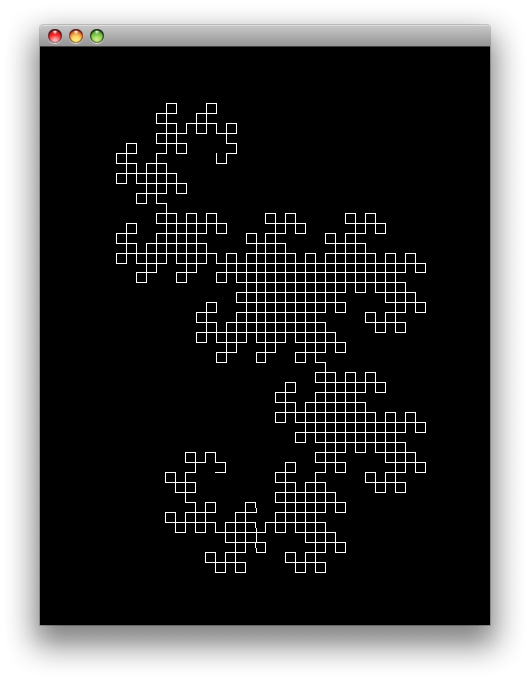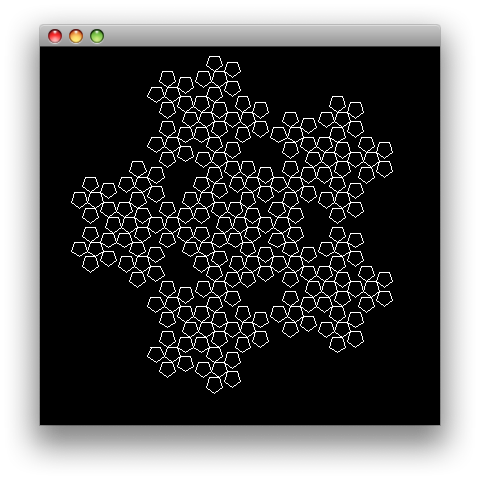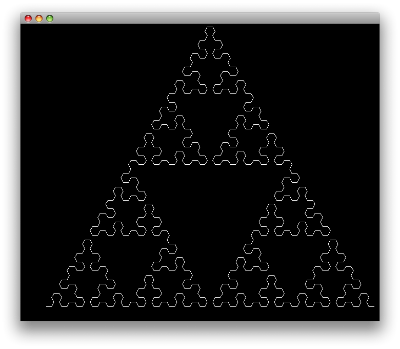## Lindenmayer System in Clojure

An L-system or Lindenmayer system is a language, which means a set of strings that is made by the application using certain rules. L-systems can be used to generate fractals such as iterated function systems.

• variables : F
• constants* : + -
• start : F
• rules : (F -> F+F-F-F+F)

Above grammar represents the Koch curve, where F means "draw forward", + means "turn left 90",and - means "turn right 90". Following is a simple implementation in Clojure to build sentences using grammars defined like this.

(ns lsystem
(:use turtle))

(defn variable? [grammer symbol]
(contains? (:variables grammer) symbol))

(defn apply-rules [grammer sentence]
(flatten
(map #(if (variable? grammer %) ((:rules grammer) %) %) sentence)))

(defn l-system [grammer n]
(loop[acc n sentence (:start grammer)]
(if (= 0 acc)
sentence (recur (dec acc) (apply-rules grammer sentence)))))


We take the axiom (start) and apply the rules n times, with each iteration we replace the variables with their corresponding rules, so the grammar above will grow such as,

• n=0: F
• n=1: F+F-F-F+F
• n=2: F+F-F-F+F+F+F-F-F+F-F+F-F-F+F-F+F-F-F+F+F+F-F-F+F

Grammar for the Koch curve in Clojure is represented like so,

(def koch-curve
{:variables #{:F}
:constants #{:+ :-}
:start [:F]
:rules {:F [:F :+ :F :- :F :- :F :+ :F]}
:actions {:F forward :+ left :- right}
:angle 90
:step 10})


There are some additions to the grammar, such as what actions will be mapped to the variables while drawing, angles for the turns and a step value to determine how much to move forward or backward.

(defn draw-system [turtle grammer sentence]
(doseq [letter sentence]
(let [action (letter (:actions grammer))]
(cond
(or (= action forward) (= action back))
(action turtle (:step grammer))
(or (= action left) (= action right))
(action turtle (:angle grammer))))))

(defn setup-turtle [turtle x y]
(pen-up turtle)
(right turtle 90)
(go turtle x y)
(pen-down turtle))


After creating a sentence for the fractal all we need to do is, iterate over the letters and command turtle to do the action that is mapped to the letter.

(def dragon-curve
{:variables #{:X :Y}
:constants #{:F :+ :-}
:start [:F :X]
:rules {:X [:X :+ :Y :F]
:Y [:F :X :- :Y]}
:actions {:F forward :+ left :- right}
:angle 90
:step 10})

(doto (turtle 450 600)
(setup-turtle -50 -200)
(draw-system dragon-curve (l-system dragon-curve 10))
(show))(def pentigree
{:variables #{:F}
:constants #{:+ :-}
:start [:F :- :F :- :F :- :F :- :F]
:rules {:F [:F :- :F :+ :+ :F :+ :F :- :F :- :F]}
:actions {:F forward :+ left :- right}
:angle 72
:step 10})

(doto (turtle 400 400)
(setup-turtle -90 -100)
(draw-system pentigree (l-system pentigree 3))
(show))(def sierpinski-triangle
{:variables #{:A :B}
:constants #{:+ :-}
:start [:A]
:rules {:A [:B :- :A :- :B]
:B [:A :+ :B :+ :A]}
:actions {:A forward :B forward :+ left :- right}
:angle 60
:step 10})

(doto (turtle 700 600)
(setup-turtle -300 -200)
(draw-system sierpinski-triangle (l-system sierpinski-triangle 6))
(show))